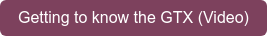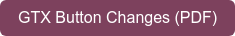# CASIO fx-83GTX & fx-85GTX

Our entry-level scientific calculator, the UK’s best-selling, has been upgraded to the latest ClassWiz technology.

The fx-83GTX and fx-85GTX replace the fx-83GT PLUS and fx-85GT PLUS. The GTX calculators are super-
easy to use while retaining all the functionality of the calculators they replace. It’s

• easier to access operations
• easier to find results
• easier to read the 4 times higher resolution display
There is also new functionality to help with calculating ratios.
The GTX range has a natural maths display and covers all the mathematical requirements for first
examinations. Check out the introductory video and the full list of functions.FeaturesSyllabus
GCSE / National and Highers / Junior and Leaving Certificate
Permitted in relevant exams
Display & Operation
Power
Resolution
High speed processing
Natural textbook display
Digit separator (integer groups of 3)
Standard form, engineering notation
Calculation
Casio input logic
Casio operation logic
Rounding – floating, decimal places, significant figures
Recurring decimal
Fraction↔recurring decimal↔decimal conversion
Number of variable stores
List of variable stored values
Prime factors
Trigonometric functions
Factorial, nCr, nPr
Random number, random integer
Logarithmic & exponential functions
Hyperbolic functions
Polar↔rectangular coordinate conversion
Statistics
Mean
Standard deviation
Quartiles
Regression (7 types)
Scrolling statistics list
Row capacity (1 variable / 2 variables)
Table
Number of functions
χ-value direct input
Ratio
Equivalent ratios
bubble_chart

# Features

## What you can do with a 83GTX & 85GTX

chevron_right### Statistics

Statistics function has been upgraded to show summary data and other useful information are a lot easier to access.### Table

You can now optionally display a table of values for 2 functions rather than just a single function. Explore values of functions by continuing the table automatically, or choose a custom value.### Useful functions

Store and recall values with greater ease. Explore prime factors and recurring decimals.

chevron_right
airplay

chevron_right